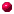# Numerical Solution of Ordinary Differential Equations Matlab Component

This page contains links to the hand-outs, problem sheets and designed for a Matlab practical on numerical methods for ordinary differential equations held in the 99/00 autumn semester at Brunel University.

## HandoutsOrganisational InformationGuide: "How to use Matlab"Problem Sheet 1Assignment 1Problem Sheet 2Assignment 2

## Solutions to Problem Sheets/Assignments

### Solutions to Problem Sheet 1Euler_One_Step.mEuler.mtest_Euler.mbetter_Euler.mtest_better_Euler.m

### Solutions to Assignment 1

#### Problem 1Euler_One_Step2.mf_example.mTesting of Euler_One_Step2

#### Problem 2new_Euler.mf_ex2.mM-file to test new_Euler

#### Problem 3The required M-file (also includes instructions for Problem 4)

#### Problem 4Runge3_One_Step.mRunge_Kutta3.mThe required M-file

### Solutions to Problem Sheet 2Euler_Step_Sys.mEuler_Sys.mTest file for Problem 2: test_Euler_Sys.mImplementation of function f : f_prob2.mImplementation of function g : g_prob2.m

### Solutions to Assignment 2

#### Problem 1Runge3_Step_Sys.m, a function called by Runge_Kutta3_SysRunge_Kutta3_Sys.mImplementation of function f : f_prob2.mImplementation of function g : g_prob2.mM-file to test Runge_Kutta3_Sys

#### Problem 2Implementation of function f : f_a2p2.mImplementation of function g : g_a2p2.mM-file to compute and plot the maximum relative errors
The answer to the final question is, that the relative error is approximately divided by eight if the number of mesh points is doubled. This is the expected behaviour of a third order method.

#### Problem 3Implementation of function f : f_a2p3.mImplementation of function g : g_a2p3.mM-file to compute the height of the spearplot of the different flight-paths (post-script)